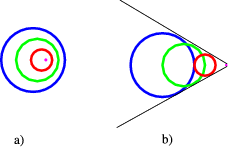$$\require{cancel}$$

2.4: Detectors

Detectors are used for various measurements on the physical processes occurring in particle physics. The most important of those are

• To identify particles.
• To measure positions.
• To measure time differences.
• To measure momentum.
• To measure energy.

Let me now go over some of the different pieces of machinery used to perform such measurements

Scintillation counters

This is based on the fact that charged particles traversing solids excite the electrons in such materials. In some solids light is then emitted. This light can be collected and amplified by photomultipliers. This technique has a very fast time response, of about 200 ps. For this reason one uses scintillators as “trigger”. This means that a pulse from the scintillator is used to say that data should now be accepted from the other pieces of equipment.

Another use is to measure time-of-flight. When one uses a pair of scintillation detectors, one can measure the time difference for a particle hitting both of them, thus determining a time difference and velocity. This is only useful for slow particles, where $$v$$ differs from $$c$$ by a reasonable amount.

Proportional/Drift Chamber

Once again we use charged particles to excite electrons. We now use a gas, where the electrons get liberated. We then use the fact that these electrons drift along electric field lines to collect them on wires. If we have many such wires, we can see where the electrons were produced, and thus measure positions with an accuracy of $$500\ \mu\text{m}$$ or less.

Semiconductor detectors

Using modern techniques we can etch very fine strips on semiconductors. We can easily have multiple layers of strips running along different directions as well. These can be used to measure position (a hit in a certain set of strips). Typical resolutions are $$5\ \mu\text{m}$$. A problem with such detectors is so-called radiation damage, due to the harsh environment in which they are operated.

Spectrometer

One uses a magnet with a position sensitive detector at the end to bend the track of charged particles, and determine the radius of the circular orbit. This radius is related to the momentum of the particles.

Čerenkov Counters

These are based on the analogue of a supersonic boom. When a particles velocity is higher than the speed of light in medium, $$v>c/n$$, where $$n$$ is the index of refraction we get a shock wave. As can be seen in Figure $$\PageIndex{1a}$$) for slow motion the light emitted by a particle travels faster than the particle (the circles denote how far the light has travelled). On the other hand, when the particle moves faster than the speed of light, we get a linear wave-front propagating through the material, as sketched in Figure $$\PageIndex{1b}$$. The angle of this wave front is related to the speed of the particles, by $$\cos\theta=\frac{1}{\beta n}$$. Measuring this angle allows us to determine speed (a problem here is the small number of photons emitted). This technique is extremely useful for threshold counters, because if we see any light, we know that the velocity of particles is larger than $$c/n$$.Figure $$\PageIndex{1}$$: Čerenkov radiation